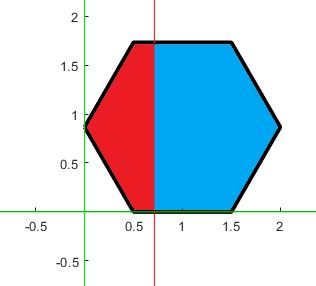# Problem 49928. Splitting Hexagon - Problem the second

Consider a hexagon sitting in Quadrant I as depicted in an example below:This hexagon is to be split into two regions (e.g., red and blue). Given the ratio between the two regions and the side of the hexagon, determine x coordinate of the line that splits the regions. The ratio between the regions (red to blue) is presented through the first two entries in the input. For example, if the ratio is 3 to 7, then these two numbers will be the first two numbers in the input. The last entry is the side of the hexagon.

### Solution Stats

35.94% Correct | 64.06% Incorrect
Last Solution submitted on Jun 10, 2023

### Community Treasure Hunt

Find the treasures in MATLAB Central and discover how the community can help you!

Start Hunting!